# Use Venn Diagrams To Verify Demorgans Law Of Complementation.

Sol:

Given:$$(A \cup B)’ = A’ \cup B'$$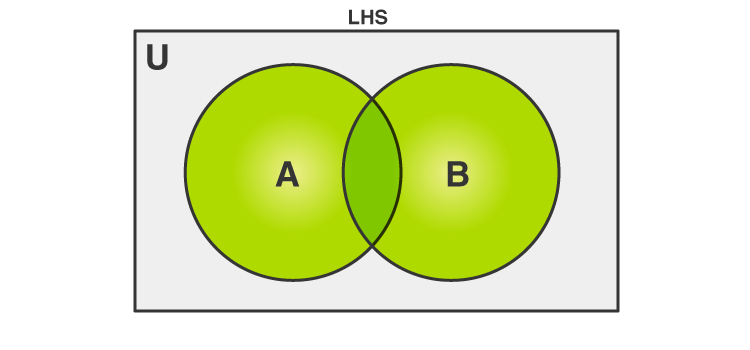The highlighted or the green coloured portion denotes A∪B. The complement of the union of A and B, i.e., (A∪B)’is a set of all those elements which are not in A∪B. This can be visualised as follows: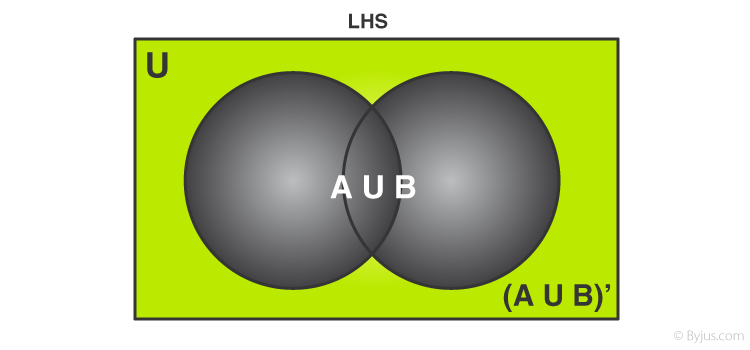Similarly, R.H.S of equation one can be represented using Venn Diagrams as well; the first part, i.e., A’ can be depicted as follows: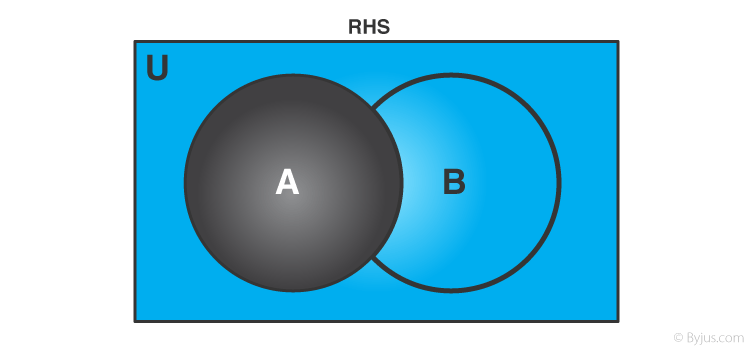The portion in black indicates set A and the blue part denotes its complement, i.e., A’.

Similarly, B’ is represented as: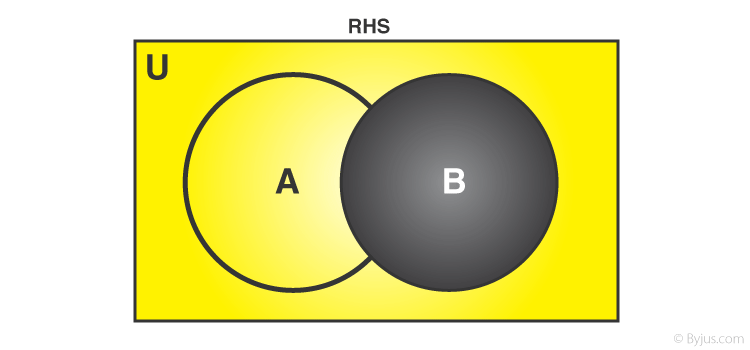The portion in black indicates set B and the yellow part denotes its complement, i.e., B’.

If fig. 3 and 4 are superimposed on one another, we get a figure similar to the complement of sets.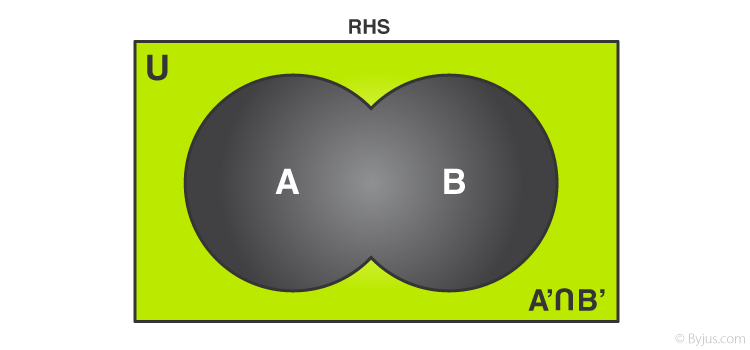According to De Morgan’s first law, the complement of the union of two sets A and B equals the intersection of the complement of the sets A and B.

$$(A \cup B)’ = A’ \cap B'$$

Explore more such questions and answers at BYJU’S.(0)(0)